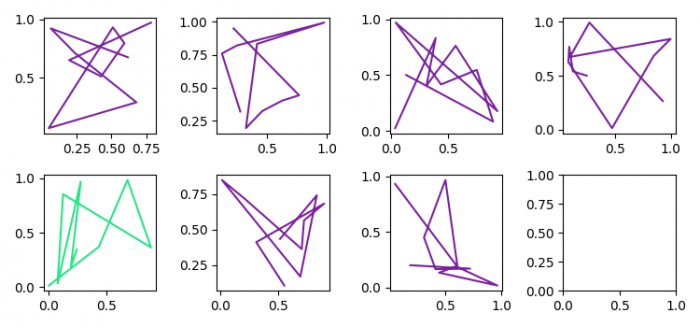# How can I dynamically update my Matplotlib figure as the data file changes?

To update a Matplotlib figure as the data file changes, we can take the following steps −

• Set the figure size and adjust the padding between and around the subplots.
• Initialize variables m and n, to get a set of subplots.
• Create a list of colors, to plot color dynamically.
• Plot dynamic data points using plot() method with random data points.
• To display the figure, use show() method.

## Example

import numpy as np
import matplotlib.pyplot as plt
import random

plt.rcParams["figure.figsize"] = [7.50, 3.50]
plt.rcParams["figure.autolayout"] = True

m = 2
n = 4

fix, axes = plt.subplots(nrows=m, ncols=n)

for j in range(6)]) for i in range(m*n)]

for i in range(m):
for j in range(n):
axes[i][j].clear()
axes[i][j].plot(np.random.rand(10), np.random.rand(10),
color=color[100 % np.random.randint(1, len(color))])
plt.pause(0.1)

plt.show()

## Output